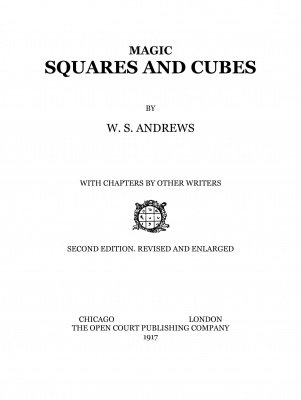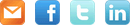search phrase

# Magic Squares and Cubes

by William Symes Andrews
\$5.00

| £3.94 | 4.35 | C\$6.74 | AUD\$7.20 | 346.00 | CN¥34.40 | JP¥537.04 | R\$19.25A classic treatise on magic squares and related arrangements of numbers.
• Publishers' Preface
• Introduction
• Chapter I. Magic Squares
• The Essential Characteristics Of Magic Squares
• Associated Or Regular Magic Squares Of Odd Numbers
• Associated Or Regular Magic Squares Of Even Numbers
• The Construction Of Even Magic Squares By De La Hire's Method
• Composite Magic Squares
• Concentric Magic Squares
• General Notes On The Construction Of Magic Squares
• Chapter II. Magic Cubes
• The Essential Characteristics Of Magic Cubes
• Associated Or Regular Magic Cubes Of Odd Numbers
• Associated Or Regular Magic Cubes Of Even Numbers
• General Notes On Magic Cubes
• Chapter III. The Franklin Squares
• An Analysis Of The Franklin Squares
• Chapter IV. Reflections On Magic Squares
• The Order Of Figures
• Magic Squares In Symbols
• The Magic Square In China
• The Jaina Square
• Chapter V. A Mathematical Study Of Magic Squares
• A New Analysis
• Notes On Number Series Used In The Construction Of Magic Squares
• Chapter VI. Magics And Pythagorean Numbers
• Mr. Browne's Square And Lusus Numerorum
• Chapter VII. Some Curious Magic Squares And Combinations
• Chapter VIII. Notes On Various Constructive Plans By Which Magic Squares May Be Classified
• The Mathematical Value Of Magic Squares
• Chapter IX. Magic Cubes Of The Sixth Order
• A "Franklin" Cube Of Six
• A Magic Cube Of Six
• Magic Cube Of Six
• Chapter X. Various Kinds Of Magic Squares
• Overlapping Magic Squares
• Oddly-Even Magic Squares
• Notes On Oddly-Even Magic Squares
• Notes On Pandiagonal And Associated Magic Squares
• Serrated Magic Squares
• Lozenge Magic Squares
• Chapter XI. Sundry Constructive Methods
• A New Method For Making Magic Squares Of Odd Orders
• The Construction Of Magic Squares And Rectangles By The Method Of "Complementary Differences"
• Notes On The Construction Of Magic Squares Of Orders In Which N Is Of The General Form 4p + 2
• Geometric Magic Squares And Cubes
• Chapter XII. The Theory Of Reversions
• Chapter XIII. Magic Circles, Spheres And Stars
• Magic Circles
• Magic Spheres
• Magic Stars
• Chapter XIV. Magic Octahedroids
• Magic In The Fourth Dimension
• Four-Fold Magics
• Chapter XV. Ornate Magic Squares
• General Rule For Constructing Ornate Magic Squares Of Orders = 0 (Mod 4)
• Ornate Magic Squares Of Composite Odd Orders
• The Construction Of Ornate Magic Squares Of Orders 8, 12 And 16 By Tables
• The Construction Of Ornate Magic Squares Of Order 16 By Magic Rectangles
• Pandiagonal-Concentric Magic Squares Of Orders 4m

1st edition 1917, 419 pages; PDF 327 pages.
word count: 72990 which is equivalent to 291 standard pages of text

 SHAREWrite your own review Click to leave a voice review or call in the US toll free 1-888-866-9150 product ID: 922483

### Frequently bought together with: